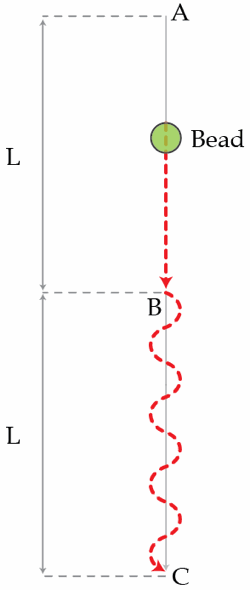# Bead on a wavy wire

A bead slides down on a frictionless wire, starting from rest at point A. The section from A to B is straight while the section from B to C is wavy (with wavelength $\lambda\ll L$). The total length of the stretched wire is $3L$. If $L=50~\text{cm}$, determine the time in seconds it takes for the bead to reach point C. Assume that the bead slides smoothly at all times.Details and assumptions

$g=9.8~\text{m}/\text{s}^2$

×

Problem Loading...

Note Loading...

Set Loading...